NCERT Solutions for Class 10 Maths Coordinate Geometry Exercise 7.2

In this page we have NCERT Solutions for Class 10 Maths Coordinate Geometry for Exercise 7.2 . Hope you like them and do not forget to like , social_share and comment at the end of the page.
Question 1 Find the coordinates of the point which divides the join of (–1, 7) and (4, –3) in the ratio 2: 3.
Question 2. Find the coordinates of the points of trisection of the line segment joining (4, –1) and (–2, –3).
Question 3. To conduct Sports Day activities, in your rectangular shaped school ground ABCD, lines have been drawn with chalk powder at a distance of 1m each. 100 flower pots have been placed at a distance of 1m from each other along AD, as shown in below figure. Niharika runs 1/4th of the distance AD on the 2nd line and posts a green flag. Preeti runs 1/5th of the distance AD on the eighth line and posts a red flag. What is the distance between both the flags? If Rashmi has to post a blue flag exactly halfway between the line segment joining the two flags, where should she post her flag?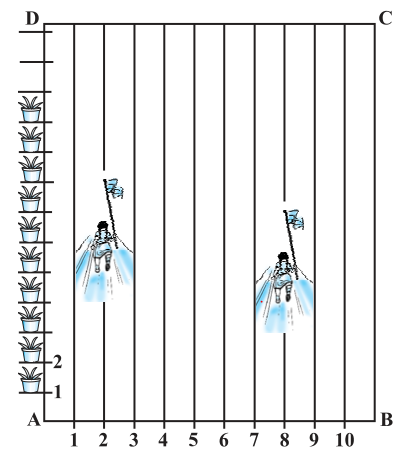Question 4. Find the ratio in which the line segment joining the points (– 3, 10) and (6, – 8) is divided by (– 1, 6).
Question 5. Find the ratio in which the line segment joining A (1, – 5) and B (– 4, 5) is divided by the x-axis. Also find the coordinates of the point of division.
Question 6. If (1, 2), (4, y), (x, 6) and (3, 5) are the vertices of a parallelogram taken in order, find x and y.
Question 7. Find the coordinates of a point A, where AB is the diameter of a circle whose centre is (2, – 3) and B is (1, 4).
Question 8. If A and B are (– 2, – 2) and (2, – 4), respectively, find the coordinates of P such that AP = (3/7) AB and P lies on the line segment AB.
Question 9. Find the coordinates of the points which divide the line segment joining A (– 2, 2) and B (2, 8) into four equal parts.
Question 10. Find the area of a rhombus if its vertices are (3, 0), (4, 5), (– 1, 4) and (– 2, – 1) taken in order.
Solution 1
Let P (x, y) be the required point. Using the section formula, we obtain.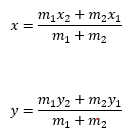So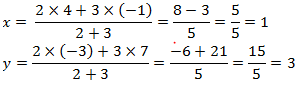Therefore, the point is (1, 3).
Solution2
Let P (x1, y1) and Q (x2, y2) are the points of trisection of the line segment joining
The given points i.e., AP = PQ = QB
Therefore, point P divides AB internally in the ratio 1:2.  And Q divides PQ in 2:1
Using the section formula, we obtain.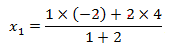=2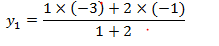=-5/3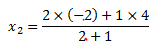=0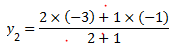=-7/3
Solution 3
It can be observed that Niharika posted the green flag at ¼ of the distance AD i.e., (1/4) ×100=25 m from the starting point of 2nd line. Therefore, the coordinates of this point G is (2, 25).
Similarly, Preeti posted red flag at 1/5 of the distance AD i.e., (1/5) ×100=20 m from the starting point of 8th line. Therefore, the coordinates of this point R are (8, 20).
Distance between these flags by using distance formula = GR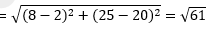The point at which Rashmi should post her blue flag is the mid-point of the line joining these points. Let this point be A (x, y). Now from mid-point section formula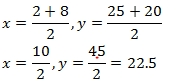Therefore, Rashmi should post her blue flag at 22.5m on 5th line
Solution 4
Let the ratio in which the line segment joining (−3, 10) and (6, −8) is divided by
point (−1, 6) be k: 1.
Then from section formulaSolving this
k=2/7
Therefore, the required ratio is 2:7
Solution 5
Let the ratio in which the line segment joining A (1, −5) and B (−4, 5) is divided by
x-axis be   k:1
Using the section formula, we obtain.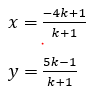Now we know that point lies on x-axis so y=0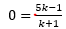Or k=1
Then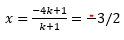So coordinates are (-3/2,0)

Solution 6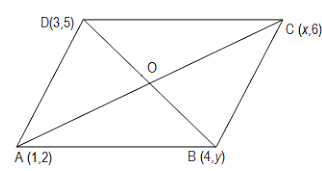Let (1, 2), (4, y), (x, 6), and (3, 5) are the coordinates of A, B, C, D vertices of a parallelogram ABCD. Intersection point O of diagonal AC and BD also divides these diagonals.
Therefore, O is the mid-point of AC and BD.
If O is the mid-point of AC, then the coordinates of O are
(1+x)/2, (2+6)/2
or (1+x)/2 ,4
If O is the mid-point of BD, then the coordinates of O are
(3+4)/2, (5+y)/2
or 7/2, (5+y)/2
Since both the coordinates are of the same point O,
So
(1+x)/2= 7/2
or x=6
(5+y)/2= 4
or y=3

Solution 7
Let the coordinates of point A be (x, y).
id-point of AB is (2, −3), which is the center of the circle.
So
(2, -3) = [(x+1)/2, (y+4)/2]
Or
(x+1)/2= 2
x=3
(y+4)/2=-3
y=-10
Therefore, coordinates are (3, -10)

Solution 8
The coordinates of point A and B are (−2, −2) and (2, −4) respectively.
Since AP = (3/7) AB
Therefore, AP: PB = 3:4
So P divides the line into the ration 3:4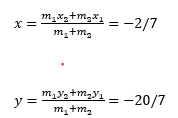Solution 9
Let P, Q, R are the point dividing line AB into four equal parts ,it can be observed that points P, Q, Rare dividing the line segment in a ratio 1:3, 1:1, 3:1 respectively.
Now coordinates of Point P will be given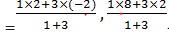= (-1,7/2)
Now coordinates of Point Q will be given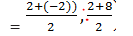= (0,5)

Now coordinates of Point R will be given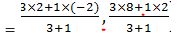= (1,13/2)
Solution 10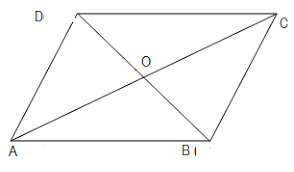Let (3, 0), (4, 5), (−1, 4) and (−2, −1) are the vertices A, B, C, D of a rhombus ABCD.
Now we know that Area of rhombus is given by
A= (1/2) d1 ×d2
Where d1 and d2 are diagonal of the rhombus
So here we just need to find the diagonals and then area can be calculated easily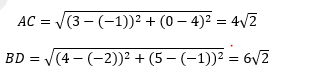So Area will be
A= (1/2) ×6√2×4√2=24

Reference Books for class 10

Given below are the links of some of the reference books for class 10 math.

You can use above books for extra knowledge and practicing different questions.

Practice Question

Question 1 What is $1 - \sqrt {3}$ ?
A) Non terminating repeating
B) Non terminating non repeating
C) Terminating
D) None of the above
Question 2 The volume of the largest right circular cone that can be cut out from a cube of edge 4.2 cm is?
A) 19.4 cm3
B) 12 cm3
C) 78.6 cm3
D) 58.2 cm3
Question 3 The sum of the first three terms of an AP is 33. If the product of the first and the third term exceeds the second term by 29, the AP is ?
A) 2 ,21,11
B) 1,10,19
C) -1 ,8,17
D) 2 ,11,20

Note to our visitors :-

Thanks for visiting our website.
DISCLOSURE: THIS PAGE MAY CONTAIN AFFILIATE LINKS, MEANING I GET A COMMISSION IF YOU DECIDE TO MAKE A PURCHASE THROUGH MY LINKS, AT NO COST TO YOU. PLEASE READ MY DISCLOSURE FOR MORE INFO.